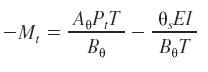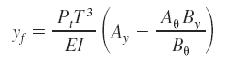Awarded as the best online publication by CIDC

When ever we are studying about a vertical pile, we need to understand that the flexural stiffness of the shaft and stiffness of the bearing soil in the upper 4D to 6D length of shaft are the two main factors on which the resistance to lateral loads of vertical pile depends.

Nondimensional solutions of Reese and Matlock help us plot the lateral-load vs. pile-head deflection relationship but the basic assumption with this is that the soil modulus K increases linearly with depth z

K= nhz
where nh coefficient of horizontal subgrade reaction.

A characteristic pile length T is given by
T=(EI/nh)1/2
where
EI= pile stiffness.

Now when a pile with free head is subjected to a lateral load Pt and moment Mt applied at the ground line, The lateral deflection y of a pile is given by

y=AyPtT3/EI+ByMtT2/EI

where
Ay and By are nondimensional coefficients.

For positive moment,
M=Am Pt T+Bm Mt

Percentage of load Transmitted To Rock Socket, Estimated by interpretation of finite-element solution for Poisson’s ratio 0.26.

 Er /Ep Er /Ep Er /Ep Ls /ds 0.25 1.0 4.0 0.5 54 48 44 1.0 31 23 18 1.5 17 12 8 2.0 13 8 4

Their is a negative moment imposed at the pile head either due to pile cap or another structural restraint, this can be calculated as a function of the head slope (rotation) from this equationwhere
theta rad represents the counterclockwise ( + ) rotation of the pile head and Atheta and Btheta are coefficients .

This value of Mt is put in previous equations to find the influence of the degrees of fixity of the pile head.

When we have a fixed-head case, the formula becomes